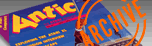`ANTIC VOL. 7, NO. 10 / FEBRUARY 1989`

# Tech Tips

BOOLEAN STICK

By Brian Murphy

It's a fairly easy task to program your joystick for drawing on the screen while avoiding Error 141 (cursor out of range). A simple program to handle such an exercise might look like this:

10 GRAPHICS 7+16
20 COLOR 1
100 S = STICK(0)
110 IF S= 7 THEN X=X+1
120 IF S = 11 THEN X = X-l
130 IF S=13 THEN Y=Y+1
140 IF S = 14 THEN Y = Y-l
150 IF S = 5 THEN X = X + l:Y = Y + 1
160 IF S = 6 THEN X = X + 1:Y = Y-1
170 IF S = 9 THEN X = X-l:Y = Y + 1
180 IF S = 10 THEN X = X-l:Y = Y-1
190 IF X>159 THEN X=159
200 IF X<0 THEN X=0
210 IF Y>95 THEN Y = 95
220 IF Y<0 THEN Y=0
230 PLOT X,Y
240 GOTO 100

But there is a much better way to code this function using Boolean Logic. You see, Atari BASIC assigns a value of one to any equation or inequality that is true and zero to any that is false. It is important to realize that you don't need the convention of an IF . .THEN statement in order to have an expression such as T=5 evaluated as true (one) or false (zero). For example:

10 T= 5
20 ? T=5

In this example the value five is assigned to T-so the expression T= 5 in line 20 is evaluated to be one. This mini-program will print a one.

Armed with this background information we're ready to rewrite the joystick function. Just replace lines 110 through 220 in the above program with the two following lines:

110 X = X + ((S = 5)+(S = 6)+(S = 7))*(x,159)-((S=9)+(S=10)+(S=11))*(X>0)
120 Y=Y+((S=5)+(S=5)+(S=9)+(S=13))*(Y<95)-((S=6)+(S=10)+(S=14))*(Y>0)

The parentheses () in the procedure are included to ensure that everything is evaluated in the proper order. Very often when using Boolean Logic you will need such parentheses. Also note that I used addition(+) to represent Logical OR and multiplication (*) for Logical AND. This is possible only if there is mutual exclusion--only one of the possible conditions can occur at any instant of time. Since a joystick can only be pointed in one direction at a time, we are assured of mutual exclusion.

Obviously this routine makes the program much shorter requiring less memory. This new coding replaces twelve lines with just two. In many cases multiple IF . .THEN statements that require separate lines can be summed up in one line.

A joystick routine is only one of many possible uses for Boolean Logic expressions. By using Boolean Logic throughout your routines you can save a great deal of RAM for that extra feature you just couldn't fit in.

AUTORUN SETUP

By Robert Wallace

Antic published a September 1988 Tech Tip called AUTOGO.BAS which creates an autorun file that will LOAD and RUN BASIC programs. But this great utility already exists and most Atari users already have it without knowing. On the DOS 2.5 master disk there is a SETUP.COM file which will create AUTORUN.SYS files for BASIC.

The menus are easy to follow. Note that SETUP.COM will work on the disk in drive 1 unless you use option 1 in the menu below:

Choose an option:

1. Change current drive number
2. Change system configuration
3. Set up AUTORUN for Boot

The DOS 2.5 Master disk came with new Atari drives from the 1050 on. If you don't have a copy, check with your local dealer or users group about the best way to get a copy.

Antic pays \$25 for every original and exclusive Tech Tip submission that we publish. Send Your 8-bit or ST disk: and printout to: Antic Tech Tips, 544 Second Street, San Francisco, CA 94107. Tech Tips welcomes very short programs that demonstrate the Atari's powers, simple hardware modifications, or useful macros for popular software.